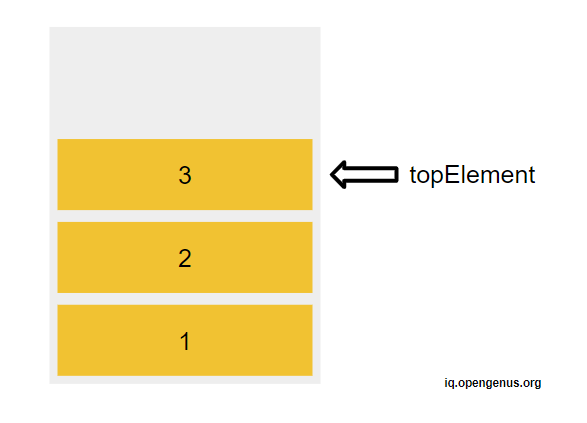# Stack Data Structure in JavaScriptIn computer science, data structures play a vital role in organizing and manipulating data efficiently. One such data structure is the stack. A stack is an abstract data type that follows the Last-In-First-Out (LIFO) principle. JavaScript, being a versatile programming language, provides built-in support for implementing stacks. In this article at OpenGenus, we will explore the stack data structure in JavaScript, understand its underlying principles, and learn how to implement and utilize stacks effectively.

• The Basics of a Stack
• The Role of the Call Stack
• Implementing a Stack Class
• Conclusion

## The Basics of a Stack

At its core, a stack is a collection of elements with two primary operations: push and pop. The push operation adds an element to the top of the stack, while the pop operation removes the topmost element from the stack. This behavior resembles a physical stack of objects, where the last item placed on top is the first one to be removed.

In JavaScript, we can implement a stack using an array or a linked list. Let's start by using an array as the underlying data structure. Consider the following code snippet:

``````const stack = [];

// Push operation
stack.push(1);
stack.push(2);
stack.push(3);

// Pop operation
const topElement = stack.pop();
console.log(topElement); // Output: 3
``````

In the above example, we create an empty stack represented by the array `stack`. We then perform three push operations, adding elements 1, 2, and 3 to the stack. Finally, we execute a pop operation, which removes the topmost element (3) and assigns it to the `topElement` variable.## The Role of the Call Stack

In JavaScript, stacks are not only used for organizing data, but they also play a crucial role in the language's execution model. JavaScript has a call stack, also known as the execution stack or program stack, which keeps track of function calls during program execution.

Every time a function is called, a new frame (also known as an activation record or stack frame) is pushed onto the call stack, storing information such as the function's arguments, local variables, and the return address. When a function completes its execution, its frame is popped from the call stack, and control returns to the previous function.

Consider the following example:

``````function foo() {
console.log("Hello from foo!");
bar();
}

function bar() {
console.log("Hello from bar!");
}

foo();
``````

In the above code, when `foo()` is called, its frame is pushed onto the call stack. Inside `foo()`, the `bar()` function is invoked, causing its frame to be added to the top of the stack. The execution order is as follows:

1. Push `foo()` frame onto the stack
2. Log "Hello from foo!"
3. Call `bar()`
4. Push `bar()` frame onto the stack
5. Log "Hello from bar!"
6. Pop `bar()` frame from the stack
7. Pop `foo()` frame from the stack

Understanding the call stack is essential for debugging and analyzing JavaScript programs. It helps developers trace the flow of execution and identify errors such as stack overflows (when the call stack exceeds its maximum capacity).

## Implementing a Stack Class

While using an array to implement a stack works perfectly fine, it can be useful to create a custom stack class with dedicated methods for push, pop, and other stack operations. This approach provides encapsulation and abstraction, making the code more readable and maintainable.

Let's create a simple Stack class in JavaScript:

``````class Stack {
constructor() {
this.items = [];
}

push(element) {
this.items.push(element);
}

pop() {
if (this.isEmpty()) {
return null;
}
return this.items.pop();
}

isEmpty() {
return this.items.length === 0;
}

size() {
return this.items.length;
}

peek() {
if (this.isEmpty()) {
return null;
}
return this.items[this.items.length - 1];
}

clear() {
this.items = [];
}
}
``````

In the above example, we define a `Stack` class with an array `items` as the underlying data structure. The class includes methods such as `push()`, `pop()`, `isEmpty()`, `size()`, `peek()`, and `clear()`, which correspond to the fundamental operations of a stack.

Using the `Stack` class, we can rewrite the previous example as follows:

``````const stack = new Stack();
stack.push(1);
stack.push(2);
stack.push(3);

const topElement = stack.pop();
console.log(topElement); // Output: 3
``````